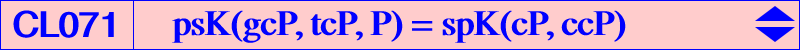Let P = p : q : r be a fixed point. M = x : y : z is a variable point and agM is the anticomplement of its isogonal conjugate. The locus of M such that P, M, agM are collinear is the cubic K(P) = psK(gcP, tcP, P) = spK(cP, ccP). Its equation is : ∑ a^2 y z (q x-r x-p y-r y+p z+q z) = 0. Properties K(P) passes through : • A, B, C where the tangents are the symmedians. • the orthocenter H of ABC. • P and gcP. • the infinite points of pK(X6, cP). The six common finite points of the two cubics lie on the isogonal transform of the line GP. • the points of pK(X6, P) on the circumcircle (O), namely A, B, C and three other points Q1, Q2, Q3 not always all real. The three other common points of the two cubics are P and two points on the line GP. Note that the tangents at Q1, Q2, Q3 to K(P) concur (at X) for all P hence K(P) is also a psK with respect to the triangle Q1Q2Q3. When Plies on the anticomplements of the cubic K652 or the deltoid H3, this point X lies on K(P) which becomes a pK in Q1Q2Q3 This occurs in particular when P = O hence K(P) = K361 with X = O, also when P = X(3146) hence K(P) = K841 with X = X(64). • the foci of the inconic with center ccP, perspector tcP. Indeed, K(P) and its isogonal transform gK(P) = spK(P, ccP) are two members of a pencil which contains pK(X6, ccP). • a given point Q ≠ H if and only if P lies on the line through Q and agQ. For instance, K(P) passes through O when P is on the Euler line. K(P) is globally invariant under two transformations 𝛗1 and 𝛗2 which are inverse of one another : for any point M on K(P), M1 = 𝛗1 (M) and M2 = 𝛗2 (M) are also two points on K(P). M1 is the tcP-Ceva conjugate of the gcP-isoconjugate of M and M2 is the gcP-isoconjugate of the tcP-Ceva conjugate of M. With M = x : y : z, this gives : M1 = (a^2 (-c^2 x y-b^2 x z+a^2 y z)) / ((q+r) x) : : and M2 = a^2 / ((q+r) x (q x+r x-p y-r y-p z-q z)) : : . *** Special cubics • K(P) is a pK if and only if it is a K0 i.e. if and only if P lies on the line GK. In this case it must contain its isopivot K and also G. These pKs belong to a same pencil generated by K002 (P = G) and K644 (P = K). See table below for other examples and also CL043. • K(P) is circular if and only if P lies on the line at infinity. The singular focus lies on the circle C(O, 2R) passing through X(399), the focus of K568 obtained when P = X(523). • K(P) is equilateral if and only if P = H giving the stelloid K028. • K(P) is a K+ (with concurring asymptotes) if and only if P lies on a cicum-cubic of the antimedial triangle passing through H (giving K028), X(20) (giving K443) and X(2889). The complement of this cubic is a circum-cubic meeting the line at infinity like pK(X6, X140) and (O) like K003 i.e. at the vertices of the CircumNormal triangle. • K(P) is a nodal (unicursal) cubic if and only if P lies ona complicated sextic passing through H (giving K028), X(145) (giving K360). The following table gives a list of catalogued cubics K(P).P K(P) remark gK(P) X(2) K002 pK K002 X(3) K361 CircumNormal cubic K026 X(4) K028 nodal stelloid K009 X(5) K849 K569 X(6) K644 pK K836 X(20) K443 K+ K376 X(30) K447 circular, F = X(10620) K446 X(69) K141 pK K177 X(81) K379 pK pK(X2092, X2) X(144) K710 contains the foci of the Mandart ellipse ? X(145) K360 Lemoine cubic K(X1) K259 X(193) K233 pK K168 X(194) K444 contains the Brocard points K512 X(385) K380 pK K357 X(511) K1134 circular, F = X(12188) K570 X(523) K568 circular, F = X(399) K567 X(524) K273 pK, circular, F = X(11258) K043 X(758) K306 circular, F = X(14663) ? X(1992) K283 pK K284 X(3091) K813 contains the X3-OAP points, see Table 53 ? X(3146) K841 K426 X(3180) K1145a pK K341a X(3181) K1145b pK K341b X(5739) K1135 pK K321 X(6515) K621 pK pK(X577, X2) X(7766) K1013 pK K1012 X(7774) K791 pK pK(X182, X2) X(7779) K354 pK K252